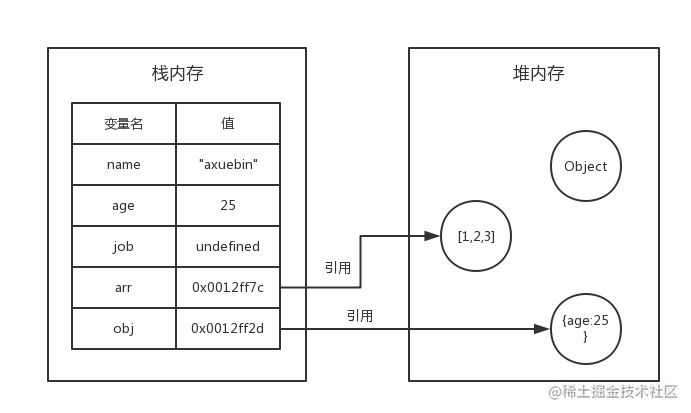# “深拷贝” 与 “浅拷贝” 的区别，JS实现深浅拷贝的几种方法

## 一、 “深拷贝” 与 “浅拷贝” 的区别

【1】javascript变量包含两种不同数据类型的值：基本类型和引用类型。

①基本类型值指的是简单的数据段，包括es6里面新增的一共是有6种，具体如下：

String、Number、Boolean、Null、Undefined、Symbol

②引用类型值指那些可能由多个值构成的对象，具体如下：

Object（Object、Array、Function）

【2】javascript的变量的存储方式--栈（stack）和堆（heap）【3】javascript值传递与址传递

``````    let a = 10; // 定义一个变量a并赋值为10
let b = a;  // 将a的值10赋值给b (a、b都是基本类型，值传递)
b++;  // b自加
console.log(a, b) // 10, 11复制代码````````````    let a = ['a', 'b', 'c']; // 定义一个数组a并赋值
let b = a;   // 数组是引用类型,采用地址传递，将a的地址赋值给b
b.push('d'); // 给b数组增加一个'd'元素
console.log(a) // ['a', 'b', 'c', 'd']
console.log(b) // ['a', 'b', 'c', 'd']复制代码``````【4】浅拷贝和深拷贝区别总结

## 浅拷贝案例

``````    // 数组的浅拷贝
let arr1 = [1, 2, 3]
let arr2 = []
for (let i in arr1) {
arr2[i] = arr1[i]
}
arr2.push(4)
console.log(arr1)  // [1, 2, 3]
console.log(arr2)  // [1, 2, 3, 4]

// 对象的浅拷贝
let obj1 = {
a: '1',
b: '2',
c: '3'
}
let obj2 = {}
for (let i in obj1) {
obj2[i] = obj1[i]
}
obj2.d = '4'
console.log(obj1) // {a: "1", b: "2", c: "3"}
console.log(obj2) // {a: "1", b: "2", c: "3", d: "4"}

// 浅拷贝函数封装
function shallowCopy(obj1, obj2) {
for(var key in obj1) {
obj2[key] = obj1[key]
}
}复制代码``````

``````    // 浅拷贝函数封装
function shallowCopy(obj1, obj2) {
for(var key in obj1) {
obj2[key] = obj1[key]
}
}

// 对象的浅拷贝
let obj1 = {
a: '1',
b: '2',
c: {
name: 'Demi'
}
}
let obj2 = {}
shallowCopy(obj1, obj2) //将obj1的数据拷贝到obj2
obj2.c.name = 'dingFY'
console.log(obj1) // {a: "1", b: "2", c: {name: 'dingFY'}}
console.log(obj2) // {a: "1", b: "2", c: {name: 'dingFY'}}复制代码``````## 深拷贝案例

``````// 深拷贝函数封装
function deepCopy(obj) {
// 根据obj的类型判断是新建一个数组还是对象
let newObj = Array.isArray(obj)? [] : {};
// 判断传入的obj存在，且类型为对象
if (obj && typeof obj === 'object') {
for(key in obj) {
// 如果obj的子元素是对象，则进行递归操作
if(obj[key] && typeof obj[key] ==='object') {
newObj[key] = deepCopy(obj[key])
} else {
// // 如果obj的子元素不是对象，则直接赋值
newObj[key] = obj[key]
}
}
}
return newObj // 返回新对象
}

// 对象的深拷贝
let obj1 = {
a: '1',
b: '2',
c: {
name: 'Demi'
}
}
let obj2 = deepCopy(obj1) //将obj1的数据拷贝到obj2
obj2.c.name = 'dingFY'
console.log(obj1) // {a: "1", b: "2", c: {name: 'Demi'}}
console.log(obj2) // {a: "1", b: "2", c: {name: 'dingFY'}}复制代码``````

## 数组的浅拷贝

【1】Array.concat()

``````    let arr = ['one', 'two', 'three'];
let newArr = arr.concat();
newArr.push('four')

console.log(arr)    // ["one", "two", "three"]
console.log(newArr) // ["one", "two", "three", "four"]复制代码``````【2】Array.slice()

``````    let arr = ['one', 'two', 'three'];
let newArr = arr.slice();
newArr.push('four')

console.log(arr)    // ["one", "two", "three"]
console.log(newArr) // ["one", "two", "three", "four"]复制代码``````## 数组的深拷贝

``````    let arr = {
a: 'one',
b: 'two',
c: {
name: 'Demi'
}
};

let newArr = JSON.parse( JSON.stringify(arr) );
newArr.c.name = 'dingFY'
console.log(arr);    // {a: "one", b: "two", c: {name: 'Demi'}}
console.log(newArr); // {a: "one", b: "two", c: {name: 'dingFY'}}

// 测试函数能否复制
let arr = {
a: 'one',
b: ()=>{
console.log('test')
}
};

let newArr = JSON.parse( JSON.stringify(arr) );
console.log(arr);    // {a: "one", b: ()=>{console.log('test')}}
console.log(newArr); // {a: "one"} // 函数没有复制成功复制代码``````

## 对象的浅拷贝

Object.assign()方法可以把任意多个的源对象自身的可枚举属性拷贝给目标对象，然后返回目标对象。但是 Object.assign() 进行的是浅拷贝

``````    let arr = {
a: 'one',
b: 'two',
c: 'three'
};

let newArr = Object.assign({}, arr)
newArr.d = 'four'
console.log(arr);    // {a: "one", b: "two", c: "three"}
console.log(newArr); // {a: "one", b: "two", c: "three", d: "four"}复制代码``````

## 浅拷贝封装方法

``````   let shallowCopy = function (obj) {
// 只拷贝对象
if (typeof obj !== 'object') return;
// 根据obj的类型判断是新建一个数组还是对象
let newObj = obj instanceof Array ? [] : {};
// 遍历obj，并且判断是obj的属性才拷贝
for (let key in obj) {
if (obj.hasOwnProperty(key)) {
newObj[key] = obj[key];
}
}
return newObj;
}复制代码``````

## 深拷贝封装方法

``````    let deepCopy = function (obj) {
// 只拷贝对象
if (typeof obj !== 'object') return;
// 根据obj的类型判断是新建一个数组还是对象
let newObj = obj instanceof Array ? [] : {};
// 遍历obj，并且判断是obj的属性才拷贝
for (let key in obj) {
if (obj.hasOwnProperty(key)) {
// 如果obj的子属性是对象，则进行递归操作,否则直接赋值
newObj[key] = typeof obj[key] === 'object' ? deepCopy(obj[key]) : obj[key];
}
}
return newObj;
}复制代码``````